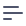# Calculating Average and Percentiles

## Use case

We want to understand the distribution of values for a certain numeric property within a dataset. We're used to average values and intuitively understand how to calculate them. However, we also know that average values can be misleading for skewed distributions which are common in the real world: for example, 2.5 is the average value for both (1, 2, 3, 4) and (0, 0, 0, 10).

So, it's usually better to use percentiles. Parameterized by a fractional number n = 0..1, where the n-th percentile is equal to a value that exceeds a specified ratio of values in the distribution. The median is a special case: it's defined as the 50th percentile (n = 0.5), and it can be casually thought of as "the middle" value. 2.5 and 0 are the medians of (1, 2, 3, 4) and (0, 0, 0, 10), respectively.

## Data schema

Let's explore the data in the Users cube that contains various demographic information about users, including their age:

[
{
"Users.name": "Abbott, Breanne",
"Users.age": 52
},
{
"Users.name": "Abbott, Dallas",
"Users.age": 43
},
{
"Users.name": "Abbott, Gia",
"Users.age": 36
},
{
"Users.name": "Abbott, Tom",
"Users.age": 39
},
{
"Users.name": "Abbott, Ward",
"Users.age": 67
}
]

Calculating the average age is as simple as defining a measure with the built-in avg type. Calculating the percentiles would require using database-specific functions. However, almost every database has them under names of PERCENTILE_CONT and PERCENTILE_DISC, Postgres and BigQuery included.

  measures: {
avgAge: {
sql: age,
type: avg,
},

medianAge: {
sql: PERCENTILE_CONT(0.5) WITHIN GROUP (ORDER BY age),
type: number,
},

p95Age: {
sql: PERCENTILE_CONT(0.95) WITHIN GROUP (ORDER BY age),
type: number,
},
},

## Result

Using the measures defined above, we can explore statistics about the age of our users.

[
{
"Users.avgAge": "52.3100000000000000",
"Users.medianAge": 53,
"Users.p95Age": 82
}
]

For this particular dataset, the average age closely matches the median age, and 95% of all users are younger than 82 years.

## Source code

Please feel free to check out the full source code or run it with the docker-compose up command. You'll see the result, including queried data, in the console.